﻿ bgods - ggplot2学习笔记之颜色标度

# ggplot2学习笔记之颜色标度

## 连续型：

``````library(reshape2)
volcano3d <- melt(volcano)
names(volcano3d) <- c("x", "y", "z")
p <- ggplot(data,aes(x,y,fill=z)) + geom_tile() p
p + scale_fill_gradient(low = 'blue', high = 'red')
``````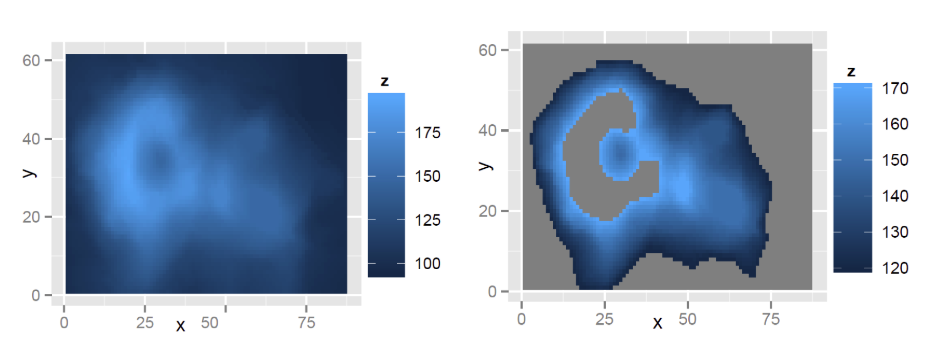第一个使用默认参数；第二图使用了参数 limits=c(120,170)，用于控制填充颜色的值范围，所以小于 120 与大于 170 的值都没有颜色（即都是灰色）；最后一个图中使用了参数 low 与 high，控制颜色在 low 与 high 之间渐变。

``````p + scale_fill_gradient2(low = 'blue', high = 'red')
p + scale_fill_gradient2(low = 'blue', high = 'red', midpoint = 150)
``````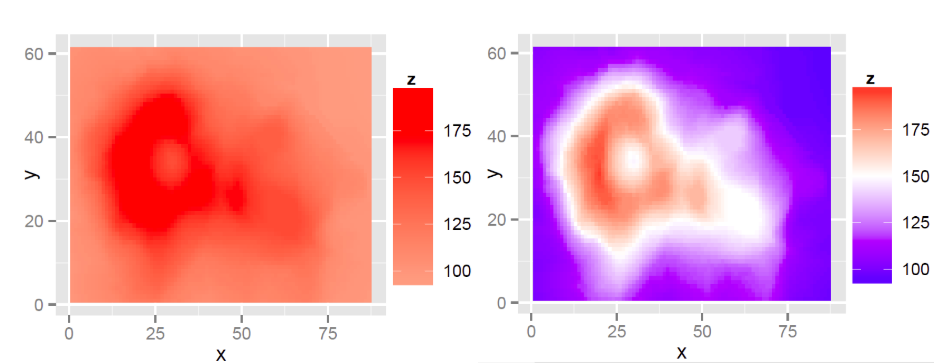以上两图都设定了颜色由 blue 到 red 渐变，但是左图使用默认参数 midpoint=0，右图使用参数midpoint=150，而 z 值的范围是 94\~195，所有他们在图中实际渐变色为，左图：浅红-红，右图：蓝-白-红 。

``````p + scale_fill_gradientn(colours = c('black','blue','red','white'))
``````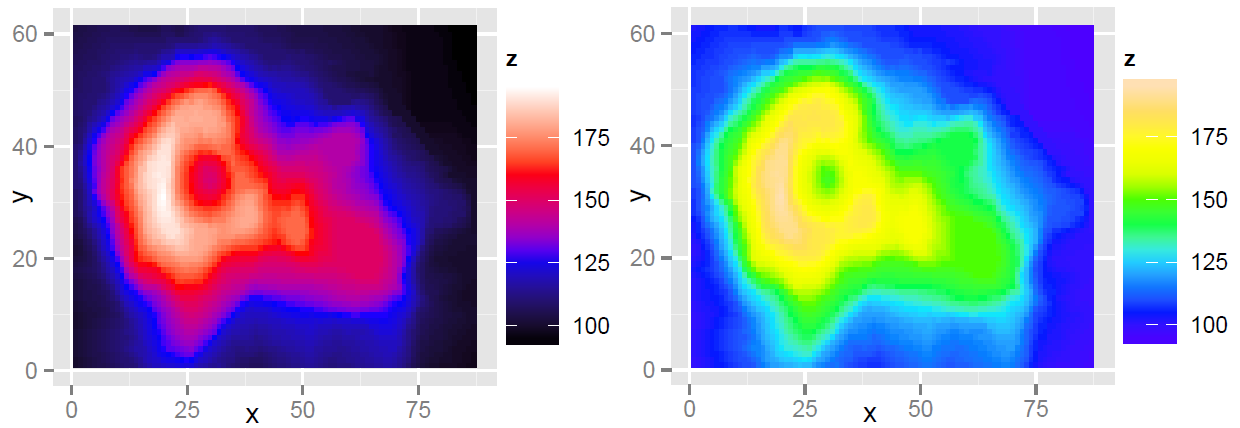## 离散型：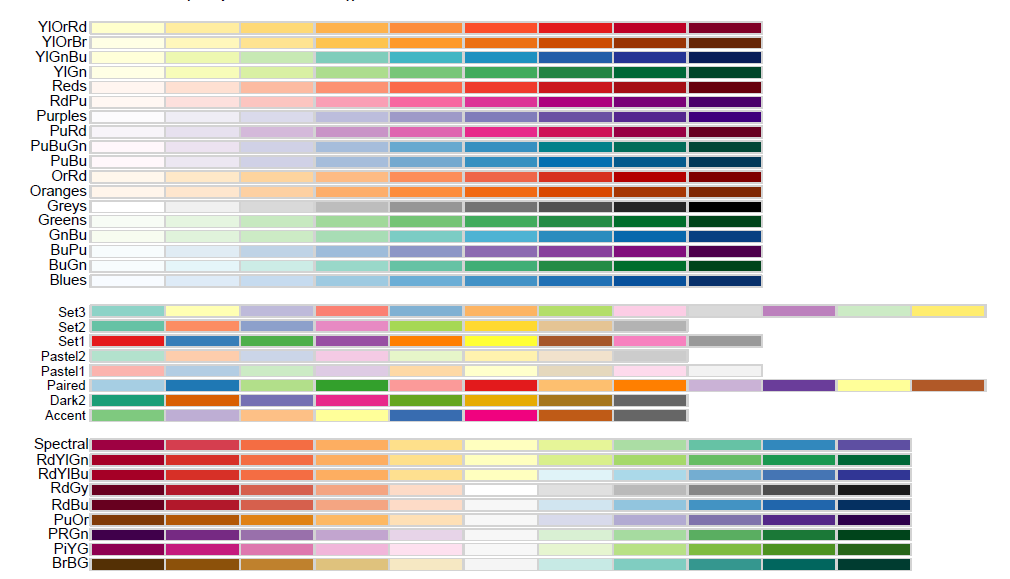``````dsamp <- diamonds[sample(nrow(diamonds), 1000), ]
(d <- ggplot(dsamp, aes(cut, price, fill = cut)) + geom_boxplot())
d + scale_fill_brewer()
d + scale_fill_brewer(palette = 2)
d + scale_fill_brewer(palette = "Spectral")
``````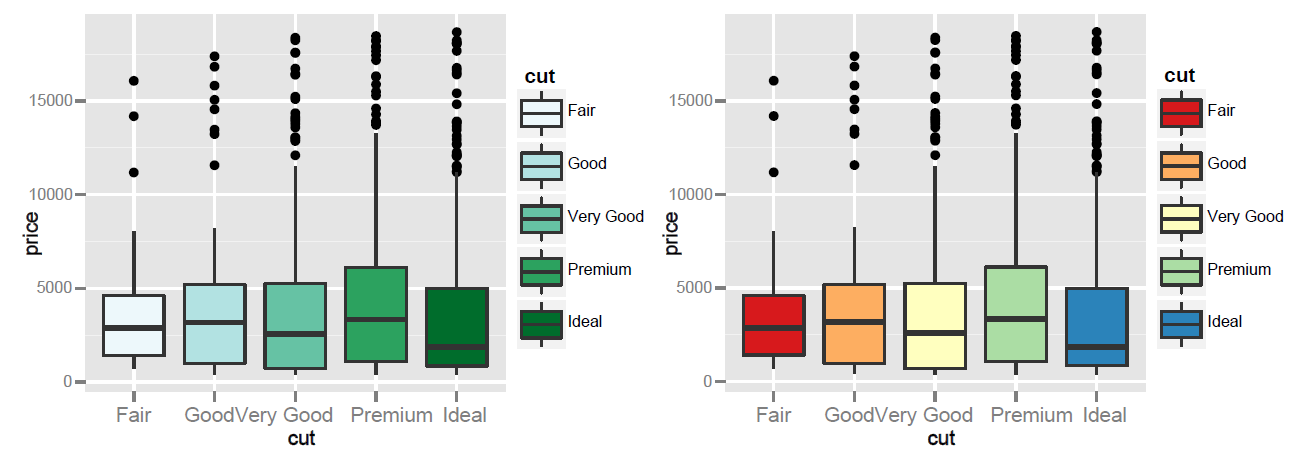左上图使用默认配色方案 cale_fill_hue()；后三种都是使用第二种配色方案，分别使用的调色板是：默认调色板、YIOrBr 调色板、Spectral 调色板。

﻿

0 评论